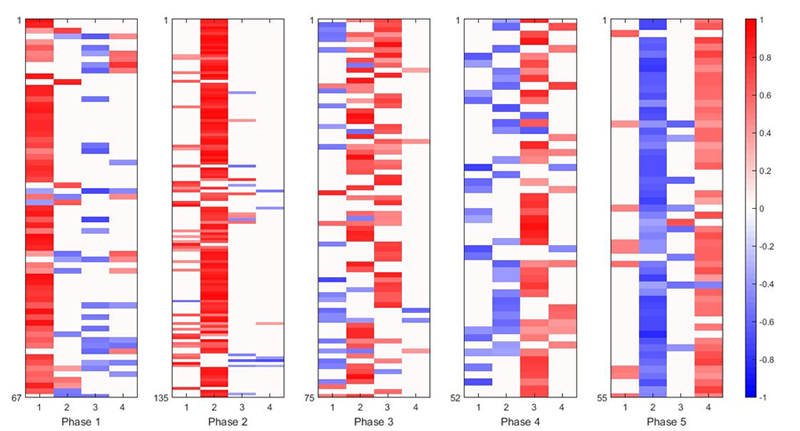Recent Studies

Studies since 2012

Notation common among several procedures

Xn-observations×p-variables column-centered data matrix

Sp-variables×p-variables sample covariance matrix

Fn-observations×m-factors score matrix, F’F = nI (identity matrix)

U:  n-observations×p-variables score matrix, UU = nI, FU = O (zero matrix)

Ψp-variables×p-variables diagonal matrix

Factor Analysis (FA)

1. Matrix Decomposition FA: Algorithm for min F, A, U, Ψ ||XFA’-UΨ||2 to obtain A and U only from S (Adachi, 2012).
2. EM Algorithm: Proving that it cannot give improper solutions (Adachi, 2013).
3. Sparse FA: min F, A, U, Ψ||XFA’-UΨ||2 s.t. Cardinality(A)=const., without using penalties (Adachi & Trendaifilov 2015).
4. Generalized Least Squares Method: min A, Ψ tr{(SAA’-Ψ2)Ψ2}2 using majorization (Adachi, 2015).
5. Caregorical FA: min F, A, U, Ψ, Q Σj||GjQjFAjUjΨj||2 s.t. QjGjGjQj = nI, GkQk being column-centered with Gk n-observations×Kj-categories membership indicator matrix (Makino, 2015).
6. Clustering FA: min F, A, Ψ nlog|Ψ2|+ tr(XGCA’)Ψ2(XGCA’)’ s.t. F = GC with G an n×g membership matrix (Uno, Satomura, & Adachi, 2016).

Principal Component Analysis (PCA)

1. Generalized Joint Procrustes Analysis: min N, F, A Σk(n1||FkNkF||2+ p1||AkNk1A||2) with XkFkAk‘=FkNkNk1Ak‘ (Adachi, 2015).
2. Sparse PCA: min F, A ||XFA’||2 over F, A s.t. Cardinality(A)=const. (Adachi & Trendaifilov 2016).
3. SparseTucker2: min F, A ||ZFH(IM’)||2 s.t. Cardinality(H)=const. with the slices of three-way data horizontally arranged in Z (Ikemoto & Adachi, 2016).

Canonical Correlation Analysis

1. Reformulation: as　maxA,B tr{XA(A’X’XA)1A’X’}{YB(B’Y’YB)1B’Y’} s.t. rank(XA)=rank(YB)≤ min(p,q) is proved, which allows oblique rotation, with [X, Y] an n × (p+q) data matrix (Satomura & Adachi, 2013).

References: clich HereResulting A in  for gene expression data

PAGE TOP